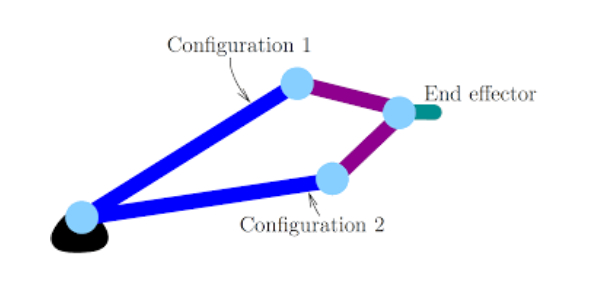# JEE Main Physics: Kinematics Set 2 Exam Quiz!

10 Questions | Attempts: 97
ShareSettings.

• 1.
Which of the following acceleration vs. time graphs represent the complete motion of an object thrown vertically upward?
• A.
• B.
• C.
• D.
• 2.
Which of the following pair of graphs represent the same motion?
• A.

1

• B.

2

• C.

3

• D.

4

• 3.
The figure shows the four paths of a ball hit by a batsman in the game of cricket. If there is no air resistance then which of the following is the correct order of the initial horizontal velocity component in decreasing order?
• A.

1>4>3>2

• B.

2>3>4>1

• C.

4>3>2>1

• D.

1>2>3>4

• 4.
A horizontally traveling bullet pierces a paper screen A and then B which are separated by a distance of 45 m. The hole made by the bulletin screen A is 20 cm above the hole in screen B. What will be the velocity of the bullet when it hits screen A? Neglect the resistance of paper and air.
• A.

450 m/s

• B.

225 m/s

• C.

332 m/s

• D.

625 m/s

• 5.
The centripetal acceleration of a particle moving in a circle of radius r is given as ac = n2rt2, where n is a positive constant. Which of the following option is correct for the above motion?
• A.

Speed of the particle =

• B.

Tangential acceleration of the particle =

• C.
• D.

None of these

• 6.
A ball is projected at an angle  with the velocity of u. What will be the average velocity between the point of projection and the highest point of the projectile?
• A.
• B.
• C.
• D.
• 7.
A ball is moving in a circle of a radius of 12 m with a speed of 2 m/s. The speed of the ball increases by 5 m/s in every one second. The total acceleration of the body after one second is:
• A.
• B.
• C.
• D.
• 8.
On the basis of the diagram which of the following statement is true:
• A.

Particle 1 will reach the ground first

• B.

All the particles will reach the ground simultaneously

• C.

All the particles will hit the ground with same speed

• D.

Only particle 2 and 3 will hit the ground with same speed

• 9.
A particle is thrown at some angle within the gravitational field of the earth with some initial velocity. Which of the following graph is not true in this context?
• A.
• B.
• C.
• D.
• 10.
An object is moving in a circular path of radius ‘R’ such that its speed ‘v’ varies as  where x is the distance and is a positive constant. What will be the acceleration of the object?
• A.
• B.
• C.
• D.

## Related TopicsBack to top
×

Wait!
Here's an interesting quiz for you.